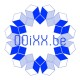Drawing the Clebsch Surface as Particleshttp://matthewarcus.github.io/polyjs/clebsch.html

https://github.com/matthewarcus/polyjs/blob/master/js/clebsch.js

Instead of using a triangulated mesh, we can display a surface in 3d by simply generating a set of random points on the surface and displaying them as a sort of particle system. Let’s do this with the famous Clebsch cubic surface: the points are stored as homogeneous coordinates, to display we multiply by a quaternion to rotate in projective space before doing the usual perspective projection to Euclidean space.

The Clebsch surface is the set of points (x0,x1,x2,x3,x4) (in projective 4-space) that satisfy the equations:

x0 + x1 + x2 + x3 + x4 = 0
x03 + x13 + x23 + x33 + x43 = 0

To simplify things, we can eliminate x0 (= x1 + x2 + x3 + x4) and rename a little, to get the single cubic equation:

(x + y + z + w)3 = x3 + y3 + z3 + w3     [***]

defining a surface in projective 3-space, with the familiar 4-element homogeneous coordinates.

Since coordinates are homogeneous, we can just consider the cases of w = 1 and w = 0 (plane at infinity), but for w = 0, it turns out the solutions are some of the 27 lines which we shall later draw separately, so for now just consider the case w = 1 for which we have:

(x + y + z + 1)3 = x3 + y3 + z3 + 1

and given values for x and y, we can solve for z easily – the cubes drop out and we just have a quadratic equation that can be solved in the usual way:

3Az2 + 3A2z + A3 - B = 0 where A = x+y+1, B = x3 + y3 + 1

We can now generate points on the surface by randomly choosing x and y and solving for z to give a set of homogeneous points (x,y,z,w) satisfying [***] and we can get further solutions by permuting the coordinates. We don’t need all permutations since some of the coordinates are arbitrary, and points that are multiples of each other are equivalent. The random points themselves are generated by this Javascript function, that generates points between -Infinity and +Infinity, but clustered around the origin.

function randpoint() {
var x = 1/(2*Math.random()-1);
if (x < 0) x -= 1;
if (x > 0) x += 1;
return x;
}

The Clebsch surface of course is famous for its 27 lines, so we draw these in as well, also as random selection of points rather than a solid line. 15 lines are points of the form (a,-a,b,-b,0) and permutations – since we are working in 4-space, this becomes 12 lines of form (a,-a,b,0) and three of form (a,-a,b,-b). These 15 lines are drawn in white and can be seen to intersect in 10 Eckardt points where 3 lines meet (though it’s hard to find a projection where all 10 are simultaneously visible). The other 12 lines are of the form (a,b,-(φa+b),-(a+φb),1) where φ is the golden ratio, 1.618.. and can be seen to form Schläfli’s “Double Six” configuration – each magenta or cyan line intersects with exactly 5 other lines, all of the opposite color.

All that remains is to project into 3-space – as usual we divide by the w-coordinate, but to get different projections, before doing this we rotate in projective space by multiplying by a quaternion & then varying the quaternion varies the projection. (Quaternion (d,-a,-b,-c) puts plane (a,b,c,d) at infinity – or alternatively, rotates (a,b,c,d) to (0,0,0,1) – it is well known that quaternions can be used to represent rotations in 3-space, but they also work for 4-space (with 3-space as a special case) – a 4-space rotation is uniquely represented (up to sign) by x -> pxq where p and q are unit quaternions). Here we multiply each point by a single quaternion to give an isoclinic or Clifford rotation – every point is rotated by the same angle.

We are using Three.js, which doesn’t seem to accept 4d points in geometries – we could write our own vertex shader to do the rotation and projection on the GPU, but for now, we do it on the CPU; updating the point positions is reasonably fast with the Three.js BufferGeometry. Actually displaying the points is simple with the THREE.Points object – we use a simple disc texture to make things a little more interesting, and attempt to color the points according to the permutations used to generate them.

The mouse and arrow keys control the camera position, square brackets move through different display modes, space toggles the rotation.

An excellent reference giving details of the construction of the surface (and good French practise) is:

http://www.mathcurve.com/surfaces/clebsch/clebsch.shtml

2 Comments on “Drawing the Clebsch Surface as Particles”

1.Wim Hespeel says:

nice work!

•matthew says:

Thanks!